Author: Oscar Cronquist Article last updated on January 08, 2019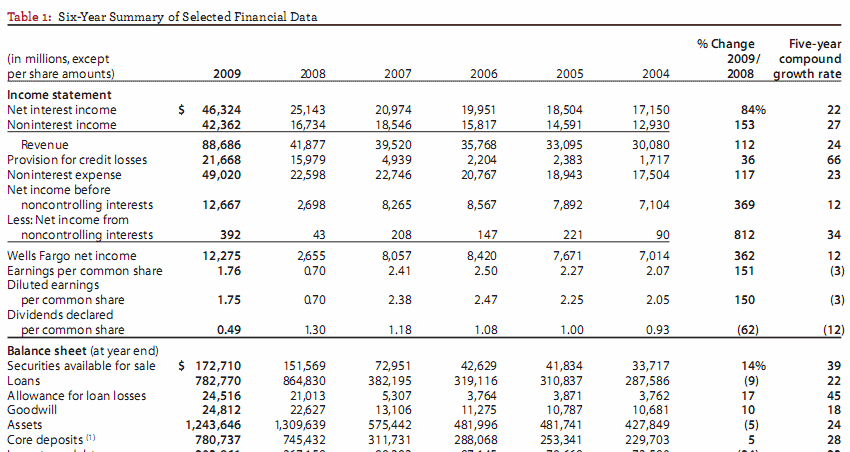This blog post describes how to insert qualifers to make "text to columns" conversion easier.

Example

I copied a table from Wells Fargo annual report (pdf), see image above. Paste it into an excel sheet.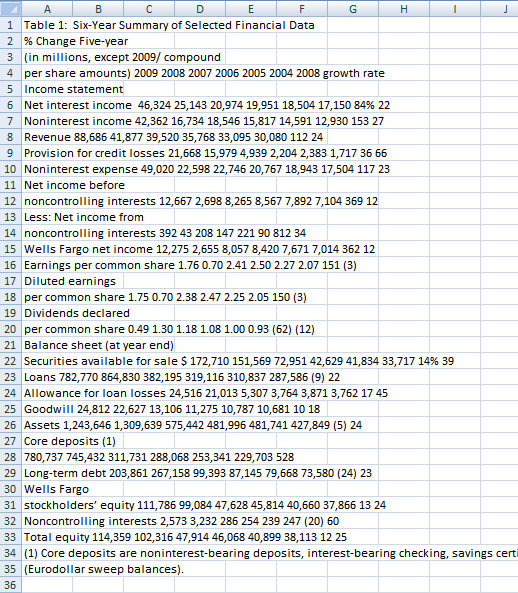I then used "Text to Columns":

1. Select column A
2. Go to tab "Data"
3. Click "Text to Columns" button.

I got the following result, see image below. Each word is split into a column each, this is not what is wanted.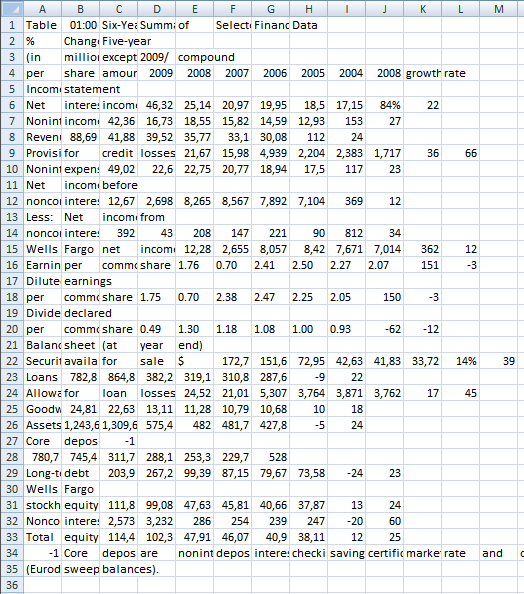The table is a mess, however, the "text to columns" wizard allows you to select a text qualifer to convert words into a single cell.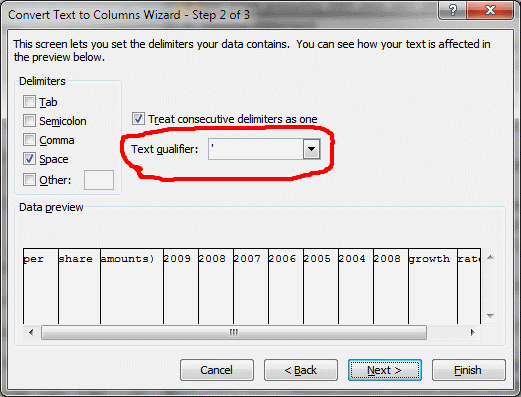This custom function inserts ' (apostrophe) before and after text. The rules are if a character is a number and the next character is a letter then insert an apostrophe before the letter.

The same thing if a character is a letter and the next character is number then insert a apostrophe before the number.

```Function Ins_text_qualifiers(Str)
Dim TLen As Long
Dim i As Long
Dim j As Long
TLen = Len(Str)Str = Trim(Str)
For i = 1 To TLen
If j = TLen + 1 Then Exit For
If Asc(Mid(Str, i, 1)) >= 65 And Asc(Mid(Str, i, 1)) <= 90 _
Or Asc(Mid(Str, i, 1)) >= 97 And Asc(Mid(Str, i, 1)) <= 122 Then
Str = Left(Str, i - 1) & "'" & Mid(Str, i, TLen)
i = i + 1
For j = i To TLen
If Asc(Mid(Str, j, 1)) >= 48 And Asc(Mid(Str, j, 1)) <= 57 Then
Str = Left(Str, j - 2) & "'" & Mid(Str, j - 1, TLen)
i = j
Exit For
End If
Next j  End IfNext i
If Asc(Mid(Str, Len(Str), 1)) >= 65 And Asc(Mid(Str, Len(Str), 1)) <= 90 _
Or Asc(Mid(Str, Len(Str), 1)) >= 97 And Asc(Mid(Str, Len(Str), 1)) <= 122 Then Str = Str & "'"
Ins_text_qualifiers = Str
End Function
```

### Example

per share amounts) 2009 2008 2007 2006 2005 2004 2008 growth rate

becomes

'per share amounts) ' 2009 2008 2007 2006 2005 2004 2008 'growth rate'

### The final result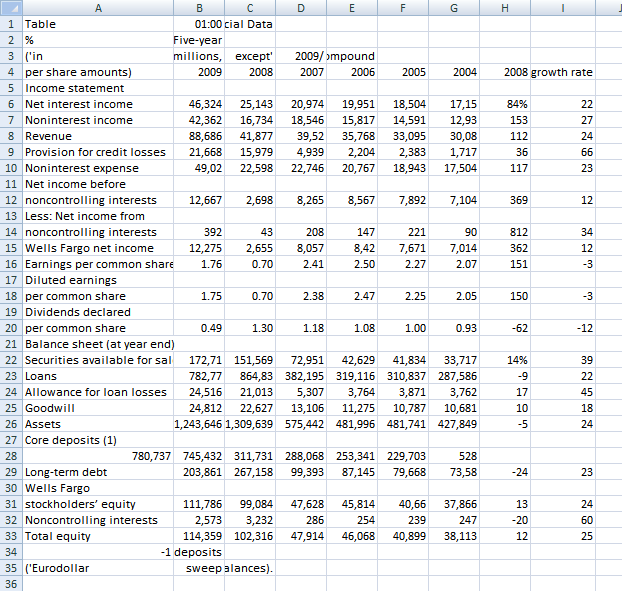It is not perfect but it is a lot better.

### How to add the user-defined function to your workbook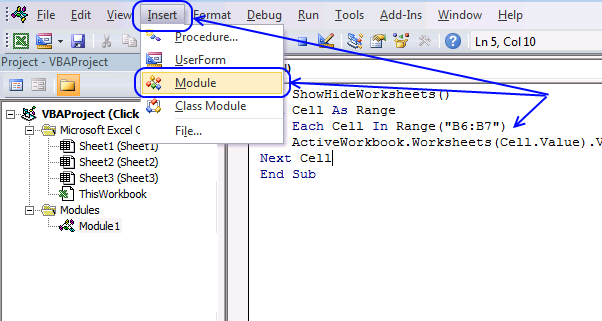(The macro shown in the image above is not used in this article, it only shows you where to paste the code.)

1. Press Alt-F11 to open the visual basic editor
2. Click Module on the Insert menu
3. Copy and paste the above user defined function
4. Exit visual basic editor
5. Select a cell (B1)
6. Type =Ins_text_qualifiers(A1) into formula bar and press ENTER

### Text to columns (Excel 2007)

1. Select "Data" tab on the ribbon
2. Click "Text to columns" button

### Download Excel filetext-qualifier1.xls